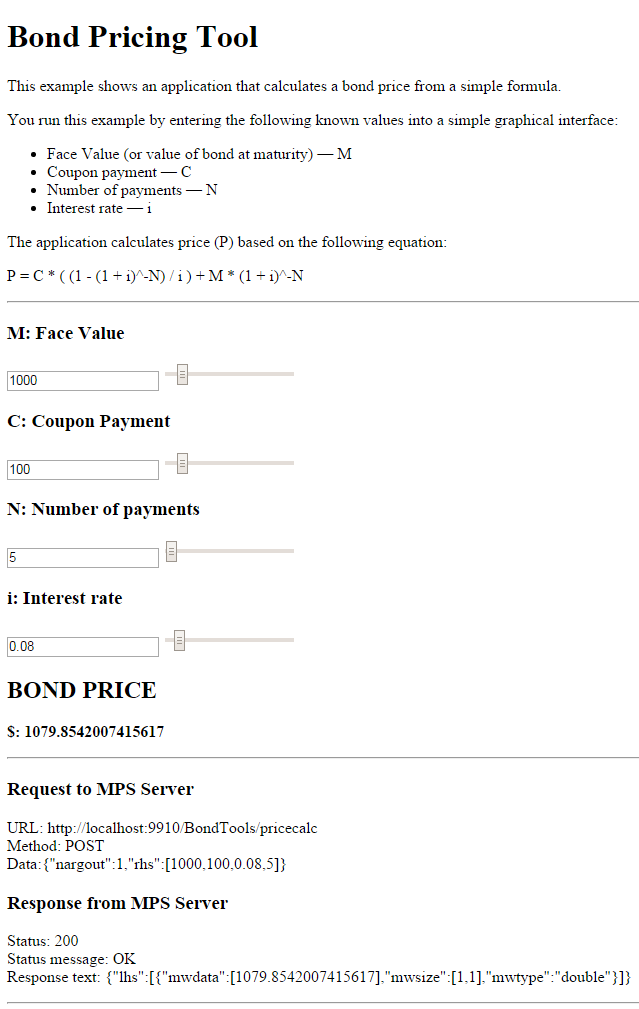## Web-Based Tool Using RESTful API, JSON, and JavaScript

This example shows how to create a web application that calculates the price of a bond from a simple formula. It uses the MATLAB® Production Server™ RESTful API and JSON Representation of MATLAB Data Types to depict an end-to-end workflow of using MATLAB Production Server. You run this example by entering the following known values into a web interface:

• Face value (or value of bond at maturity) — `M`

• Coupon payment — `C`

• Number of payments — `N`

• Interest rate — `i`

The application calculates price (`P`) based on the following equation:

`P = C * ( (1 - (1 + i)^-N) / i ) + M * (1 + i)^-N`
Use the sliders in the web application to price different bonds.

### Step 1: Write MATLAB Code

Write the following code in MATLAB to price bonds. Save the code using the filename `pricecalc.m`.

```function price = pricecalc(face_value, coupon_payment,... interest_rate, num_payments) M = face_value; C = coupon_payment; N = num_payments; i = interest_rate; price = C * ( (1 - (1 + i)^-N) / i ) + M * (1 + i)^-N;```

### Step 2: Create a Deployable Archive with the Production Server Compiler App

To create the deployable archive for this example:

1. On the Apps tab, select the Production Server Compiler App.

2. In the Application Type list, select Deployable Archive.

3. In the Exported Functions field, add `pricecalc.m`.

4. Under Archive information, change `pricecalc` to `BondTools`.

5. Click .

The generated deployable archive, `BondTools.ctf` is located in the `for_redistribution` folder of the project.

### Step 3: Place the Deployable Archive on a Server

2. Create a server using `mps-new`. See Create Server Instance for more information. If you have not already setup your server environment, see `mps-setup` for more information.

3. If you have not already done so, specify the location of the MATLAB Runtime to the server by editing the server configuration file `main_config` and specifying a path for `--mcr-root`. See Configure Server for details.

4. Start the server using `mps-start`, and verify it is running with `mps-status`.

5. Copy the `BondTools.ctf` file to the `auto_deploy` folder on the server for hosting.

### Step 4: Enable Cross-Origin Resource Sharing (CORS) on the Server

Enable Cross-Origin Resource Sharing (CORS) by editing the server configuration file, `main_config` and specifying the list of domain origins from which requests can be made to the server. For example, setting the `cors-allowed-origins` option to `--cors-allowed-origins *` allows requests from any domain to access the server. See `cors-allowed-origins` and Configure Server for details.

### Step 5: Write JavaScript Code using the RESTful API and JSON

Write the following JavaScript® code using the RESTful API and JSON Representation of MATLAB Data Types as a guide. Save this code as a JavaScript file named `calculatePrice.js`.

Code:

### Step 6: Embed JavaScript within HTML Code

Embed the JavaScript from the previous step within the following HTML code by using the following syntax:

```<script src="calculatePrice.js" type="text/javascript"></script>```

Save this code as an HTML file named `bptool.html`.

Code:

### Step 7: Run Example

Confirm that the server with the deployed MATLAB function is running. Open the HTML file `bptool.html` in a web browser. The default bond price is `NaN` because no values have been entered as yet. Try the following values to price a bond:

• Face Value = \$1000

• Coupon Payment = \$100

• Number of payments = 5

• Interest rate = 0.08 (Corresponds to 8%)

The resulting bond price is \$1079.85.

Use the sliders in the tool price different bonds. Varying the interest rate results in the most dramatic change in the price of the bond.## SupportGet trial now